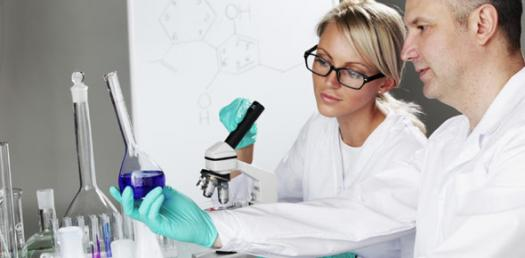# CBSE Class 12th Chemistry Practice Paper Set-I

50 Questions | Total Attempts: 864SettingsThis practice paper is completely based on the CBSE class 12thChemistry question paper 2013 in MCQ form to build you concept in class 12thChemistry This practice paper can also be helpful for JEE (AIEEE) and NEET (AIPMT) For example: Q. When R is an alkyl group  exist but  doesn’t. Give reason The above given question could be asked in JEE (AIEEE) and NEET (AIPMT) in the following way:   Q. When R is an alkyl group   exist but   doesn’t. Because of: Presence of f-orbital Absence of d-orbital Presence of d-orbital Absence of f-orbital Learn and judge yourself with jagranjosh. Com practice question paper that how question are being framed in CBSE class 12th board exam as

• 1.
Which of the following phenomenon has a higher enthalpy of adsorption?
• A.

Physisorption

• B.

Chemisorption

• C.

Desorption

• D.

None of these

• 2.
Name the method used for refining ofcopper metal.
• A.

Electrolytic method

• B.

Vapour phase refining

• C.

Zone refining

• D.

Liquation

• 3.
Which of the following gases cannot be prepared from chlorine gas?
• A.

Phosgene gas

• B.

Tear gas

• C.
• D.

Carbon dioxide

• 4.
Write the IUPAC name ofthe following compound:
• A.

2-chloro-3,3-dimethylbutane

• B.

3-chloro-2,2-dimethylbutane

• C.

2-chloro-2,2-dimethylbutane

• D.

3-chloro-3,3-dimethylbutane

• 5.
Which of the following molecule has highest boiling point?
• A.
• B.
• C.
• D.
• 6.
What are the products of hydrolysis of sugar?
• A.

Sucrose

• B.

Sucrose & Glucose

• C.

Glucose

• D.

Maltose

• 7.
Given molecule is a?
• A.

Copolymer

• B.

Homopolymer

• C.

Both A & B

• D.

None of these

• 8.
Which of the following shows Schottkydefects?
• A.

Metals

• B.

Covalent

• C.

Ionic

• D.

None of these

• 9.
Which of the following defects does not alter the density of related solids?
• A.

Schottky defect

• B.

Vacancy defect

• C.

Frenkel defect

• D.

Interstitial defect

• 10.
Which of the following atoms increase the Conductivity of silicon?
• A.

P

• B.

Al

• C.

Ga

• D.

In

• 11.
Aluminium crystallizes in an FCC structure. Atomic radius of the metal is 125 pm. what is the length of the side of the unit cell of the metal?
• A.

355.5 pm

• B.

353.5 pm

• C.

553.5 pm

• D.

535.5 pm

• 12.
The standard electrode potential (E°) for Daniell cell is + 1.1 V. Calculate for the reaction:
• A.

212.3 KJ / mol

• B.

-215.3 KJ / mol

• C.

-222.3 KJ / mol

• D.

-212.3 KJ / mol

• 13.
For a reaction A + B P , the rate law is given by:  What is the order of this reaction?
• A.

2

• B.

1/2

• C.

2.5

• D.

1

• 14.
A first order reaction is found to have a rate constant k = 5.5 x 10-14s-1. Find the half life of the reaction?
• A.

• B.
• C.
• D.
• 15.
Name the method used for removing gangue from sulphideores
• A.

Gravity separation

• B.

Hydraulic washing

• C.

Magnetic separation

• D.

Froth floatation

• 16.
Which of the following is the purest form of iron?
• A.

Cast iron

• B.

Wrought iron

• C.

Steel

• D.

None of these

• 17.
What is the hybridisation of XeOF4?
• A.
• B.
• C.
• D.
• 18.
Complete the following:
• A.
• B.
• C.
• D.
• 19.
In the mechanism of the following reaction: Which of the following is a fast step reaction?
• A.

Formation of carbocation

• B.

Formation of protonated alcohol

• C.

Removal of proton & formation of ethene

• D.

None of these

• 20.
Identify thegiven reaction?
• A.

Williamson ether synthesis

• B.

Gattermann Koch reaction

• C.

Reimer-Tiemann reaction

• D.

None of these

• 21.
In Williamson ether synthesis, attack of alkoxide ion follows which pathway?
• A.

SN2

• B.

SN1

• C.

E2

• D.

E1

• 22.
Which of the following is a thermosetting polymer?
• A.

Urea

• B.

PVC

• C.

Polythene

• D.

Paper

• 23.
Which of the following becomes hard on heating?
• A.

Polythene

• B.

PVC

• C.

Paper

• D.

Formaldehyde

• 24.
The rate of a reaction becomes four times when the temperature changes from 293 K to 313 K. Calculate the energy of activation (Ea) of the reaction assuming that it does not change with temperature. [R = 8.314 J/Kmol, log 4 = 0.6021
• A.

50.86 Kj / mol

• B.

52.86 Kj / mol

• C.

51.86 Kj / mol

• D.

42.86 Kj / mol

• 25.
Gold sol is an example of?
• A.

Lyophobic sols

• B.

Macromolecular colloids

• C.

Macromolecular colloids

• D.

Emulsions

Related TopicsBack to top